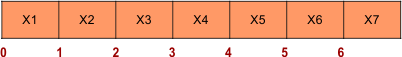Select Page

# Producer Consumer Problem

There are two processes in Producer consumer problem. One is the PRODUCER and other is the CONSUMER process. PRODUCER produces the items and CONSUMER consumed it.

These both processes are running parallel and sharing some resources like main memory. That’s why, these are also known as co-operative processes.  But the problem is that, these processes are not synchronized.

## Producer Consumer Problem With Example:

In producer section, producer produces and item and put it into buffer which is a memory and consumer take out the item from buffer. In this way buffer memory is used as shared memory in these two processes.

The explanation of Producer Consumer Problem program in C language is.

```Int COUNT = 0; // Shared variable tells how many items are there in the memory
Int N=10 // Shared variable where N (0-9) is the size of buffer.```

```Void Producer (void) {
Int IN =0 // local Variable that works as pointer Int itemP // Create an local integer Variable ItemP While(true) //condition always true    {Produce_item(itemP); // function for which item to produce i.e. pass X1 value in function to produce item X1.
While(COUNT ==N); // Buffer is full ( process go to infinite loop, if condition is false then program next execute) Buffer[IN] = itemP; // place item in buffer location 0 IN = (IN+1) MOD  N; // IN Moves to Next empty slot in buffer.
Count = Count +1; // count value incremented by 1 (because one item is produced in buffer)
} }```

```Void Consumer(void) {
Int OUT =0 // local Variable that works as pointer
Int itemC // Create an local integer Variable ItemC While(true) //condition always true
{While(COUNT ==0);  ( process go to infinite loop, if condition is false then program next execute)
Item C = Buffer(OUT); // Buffer location value stored in itemC OUT= (OUT+1) MOD N; // OUT Moves to Next filled slot in buffer
Count = Count -1; // count value decremented by 1 (because one item is consumed in buffer) Execute_item(itemC); // further processing on consumed item
}   }```

Buffer (Memory)Where 0 to 6 are different locations of memory. And X1, X2 are items produced by producer Code. Here we test the producer-consumer problem through different cases.

### CASE 01:

When a producer produces an item by executing producer code and consumer consumes that item by executing consumer code. Make sure there is no interrupt occur while execution.  Then there will be no problem with that code. Producer consumer code works perfect.

### CASE 02:

Let we are executing the producer code. buffer already contains 5 items . So, COUNT = 5. Now CPU wants to execute the following instruction to produce 6th item in producer code.

COUNT =COUNT +1

To execute above instruction CPU has to execute three micro-instructions given below,

```LOAD R1, M[COUNT] // Load the value of COUNT in Register( R1) from COUNT, So R1=5
INCR[R1] // increment the Register ( R1) value by 1 So, R1=6
STORE M[COUNT], R1 // Save the R1 value in Memory```

Suppose an interrupt is occurring just after the execution of second micro-instruction, in Producer section as

```LOAD R1, M[COUNT]
INCR[R1]
Any Interrupt occur
STORE M[COUNT], R1```

Till here, the value of R1 is incremented to 6 because (COUNT =5). But it still not loaded in main memory. Still, COUNT= 5 in main memory.  Execution-record of this process is saved in its PCB by OS. And CPU control preempt to Consumer code.

Now, when CPU is executing the following instruction in consumer section then,

COUNT = COUNT -1

To execute above instruction, again CPU has to execute again three micro-instructions.

```LOAD R2, M[COUNT] // Load the value of COUNT in Register( R2) from COUNT , So R1=5
DECR [R1] // R1 decremented by 1, So R1=4
STORE M[COUNT], R2 // Save the R2 value in COUNT  Memory```

And let suppose again an interrupt is occur just after the second micro-instruction, in consumer section

```LOAD R2, M[Count]
DECR [R2]
Any interrupt occur
STORE M[Count], R2```

In this way, the value of R2 becomes 4. It is because of minus 1 in R2. But R2 still not save in main memory. Still, COUNT= 5 in main memory. Then CPU again preempted to producer code. Then OS store the record of this process in its PCB and CPU shift to Producer section to execute remaining code. Now it starts from third micro-instruction for execution.

As CPU Execute the third instruction of consumer code the value of COUNT variable becomes 6 and it stored in main memory because OS resume the process instead of restart.

After executing the producer section, CPU pre-empt back to consumer section to execute remaining code start from third micro-instruction. Now, COUNT variable value becomes 4 and stored in main memory.

## Instruction execution Series: Producer Instruction1, instruction2 Consumer Instruction1, instruction2 Producer instruction3 Consumer Instruction3.

### Conclusion:

As CPU execute the both process parallel. As in above example, process P1 is incremented by 1 and COUNT variable becomes +1. P2 is decremented by and 1 COUNT variable becomes -1 . So, The Result should be 5 (5+1-1=5) but the value of COUNT in main memory is 4 which is incorrect.

Producer Consumer problem can be overcome by semaphore, monitor and Paterson Solution etc.

Note: Reason of CPU preempt are

• interrupt
• I/O call

Help Other’s By Sharing…